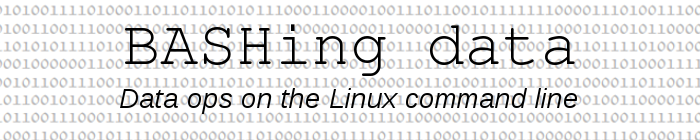For a full list of BASHing data blog posts see the index page.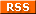# How to use flags in AWK (revisited)

I first published this article in 2016 on the Linux Rain website. The post was updated here so it could be included in the archive of BASHing data posts (and A Data Cleaner's Cookbook) in Zenodo.

Flags in AWK are variables which are set to either true or false. They're handy for defining ranges over which AWK can act, as shown below. The AWK used here is GNU AWK 4 (gawk 4).

Sometimes flags aren't needed. I'll demonstrate with a simple text file called "demo", which has 6 lines with 3 comma-separated letters on each line:

a,b,c
b,d,b
c,j,k
x,e,d
s,r,x
m,n,o

Here are 3 operations on "demo" which don't require flags:

Print the line with 'j' as second letter:
awk -F"," '\$2=="j"' demo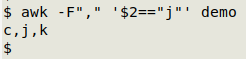Print all lines up to, but not including, the line with "j" as second letter:
awk -F"," '\$2=="j" {exit} 1' demo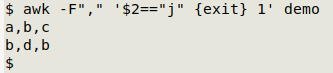When the pattern \$2=="j" is matched, the program exits. The "1" at the end of this command tells AWK to print every line it processes. It's AWK shorthand for if 1 is true, print the line, and "1" is always true (1 always equals 1).

Print all lines up to and including the line with "j" as second letter:
awk -F"," '\$2=="j" {print; exit} 1' demo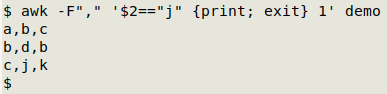When the pattern \$2=="j" is matched the line is printed, then the program exits.

Flag on. Continuing with "demo", here are some simple uses for a flag:

Print all lines starting with the line that has "j" as second letter:
awk -F"," '\$2=="j" {f=1} f' demo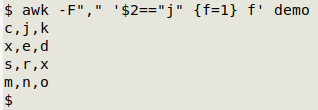When the pattern \$2=="j" is matched, the flag "f" is "turned on: the variable "f" is set equal to 1, meaning "f" is true. The flag doesn"t have to be called "f". It can be called "chrysanthemum" or "holysmoke" or "qqqqq" or "x" or any other simple string.

The "f" at the end is AWK shorthand again, like the "1" used above. It means if "f" is true, print the line. Since the flag was turned on earlier — when the pattern \$2=="j" was matched in the line c,j,k — the current line is printed.

Print all lines starting just after the line with "j" as second letter:
awk -F"," '\$2=="j" {f=1; next} f' demo
awk -F"," 'f; \$2=="j" {f=1}' demo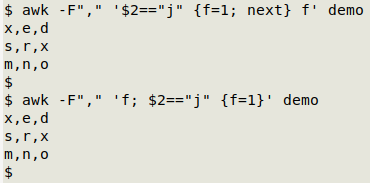In the first command, the flag is turned on when the line c,j,k is read, but then the next command tells AWK to drop whatever it's doing and move to the next line, so the "f" at the end of the command isn't acted upon and c,j,k doesn't get printed.

An alternative is the second command. Here the first instruction tells AWK to print the line if the flag "f" is on. When the c,j,k line is reached, the flag isn't yet on and the line isn't printed. The flag is only turned on after the pattern \$2=="j" is matched.

Flag on, flag off. Still with "demo", some commands that involve unsetting a flag:

Print all lines from the first line with "c" as third letter to the first line with "s" as first letter, inclusive:
awk -F"," '\$3=="c" {f=1} \$1=="s" {print; f=0} f' demo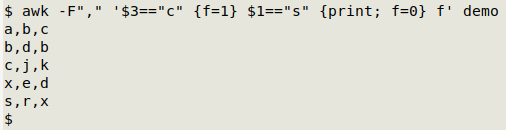The flag is turned off (unset) when the s,r,x line is read, so the last line of "demo" (m,n,o) isn't printed.

Print all lines from the first line with "c" as third letter up to, but not including, the first line with "s" as first letter:
awk -F"," '\$3=="c" {f=1} \$1=="s" {f=0} f' demo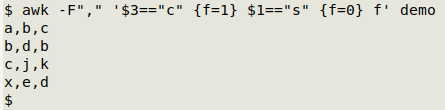The line with s,r,x isn't printed because the flag is turned off before AWK is told to print the line if the flag is true.

Print all lines between the first line with "c" as third letter and the first line with "s' as first letter:
awk -F"," '\$3=="c" {f=1; next} \$1=="s" {f=0} f' demo
awk -F"," '\$1=="s" {f=0}; f; \$3=="c" {f=1}' demo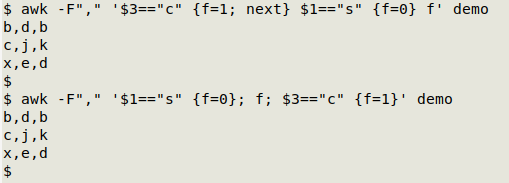The first command follows the rules demonstrated above. The second command looks a little strange at first but is very logical. The \$3=="c" line doesn't get printed because when AWK processes it, the instruction to print a line when the flag is on (f) appears before the flag has been turned on ({f=1}). The next 3 lines get printed because the flag is on. The \$1=="s" line doesn't get printed because the flag is turned off ({f=0}) before AWK sees the instruction f.

On/off, on/off. Flags can be turned on and off repeatedly as AWK processes a file. For a demonstration, here's a list of fruit names in a file called "fruit":

pear
apple
cherry
orange
lemon
raspberry
apple
loquat
feijoa
orange
loquat

Print all lines from "apple" to "orange", inclusive:
awk '/apple/ {f=1} /orange/ {print; f=0} f' fruit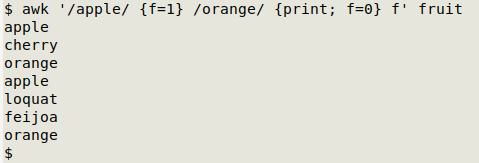Print all lines from "apple" to "orange", but not including "apple" or "orange":
awk '/orange/ {f=0}; f; /apple/ {f=1}' fruit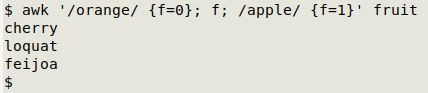Counting the on/off's. Still with "fruit", two ingenious commands based on a suggestion from developer waldner:

Print the lines between the first "apple" and "orange", but not the second, and vice-versa:
awk '/orange/ {f=0}; f && c==1; /apple/ {f=1; c++}' fruit
awk '/orange/ {f=0}; f && c==2; /apple/ {f=1; c++}' fruit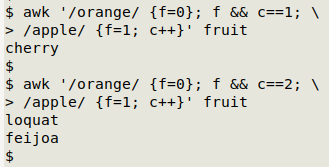To understand how that first command works, it helps to follow AWK as it reads "fruit" one line at a time.

The first line (pear) doesn't match apple or orange and the flag isn't on, so AWK does nothing.

The second line (apple) doesn't match orange and the flag isn't on, so AWK ignores the first and second instructions in the command. The line matches apple, so AWK turns on the flag and sets a counter variable "c" and starts incrementing it from 1 (the default starting number for a counter in AWK). No printing yet.

The third line is cherry. The flag is on and the counter reads 1 (for the 1 apple found so far), so the line gets printed, following the instruction f && c==1.

The fourth line is orange. The flag is turned off, and nothing gets printed by the second instruction in the command.

Nothing for AWK to match or do with the next 2 lines, lemon and raspberry, since the flag is off.

Now another apple line and the flag is turned on again and the counter gets incremented to 2. Although the flag is on, none of the following lines get printed because the counter is at 2, and printing only happens when the counter is at 1.

The second command has a similar logic, except that printing only happens when the counter is at 2.

A two flags trick. The flag commands shown above are OK for finding lines between a first starting pattern and a first ending pattern. If the situation is more complicated, as in this list of fruit names (a file named "tricky"), things get tricky:

pear
apple
apple
cherry
orange
orange
lemon
raspberry
apple
strawberry
apple
loquat
feijoa
orange
loquat

The usual commands won't work for finding just the names between the closest-occurring "apple" and "orange". For example: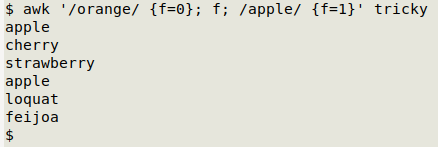AWK has followed its instructions, and returned both the second "apple" in line 3 and the "strawberry" and "apple" in lines 9 and 10. To get just the names between the closest-occurring "apple" and "orange", two flags can be used:
awk '/orange/ {f=g=0}; f && g; /apple/ && !f {f=1; next}; /apple/ && f {g=1}' tricky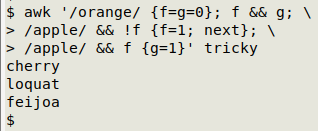Here a line is printed only if both flags, f and g, are on. Note that this particular trick will suit this particular file, but it isn't a general solution. Two general solutions were offered by AWK wizards Ed Morton and "pk" when I posted the problem on the comp.lang.awk forum. As applied to "tricky", both solutions accumulate lines between "apple" and "orange" in a variable. Here's Morton's solution:
awk '/orange/ {if (f) printf "%s", buf; f=0}; f {buf=buf \$0 ORS}; /apple/ {buf=""; f=1}' tricky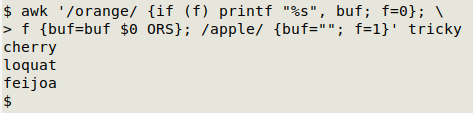If "apple" is matched, a flag is turned on and the "buf" variable is emptied. After "apple" has been matched, the next lines (not matching "orange" or "apple") are added to "buf" because f is true, and are separated with the output record separator (ORS, here a newline). If "orange" is matched and f is true (because it has been preceded by "apple"), the contents of "buf" are printed and the flag is turned off.

The general solution from "pk" looks like this as applied to "tricky" (split over two lines for clarity):
awk '/apple/ {f=1; b=s=""; next}; /orange/ && f {f=0; print b; b=s=""; next}; \
f {b=b s \$0; s=RS}' tricky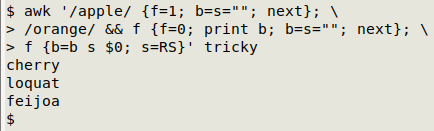This works like Morton's solution, but uses a different order of instructions and sets the record separator as a variable.

Last update: 2020-10-21
The blog posts on this website are licensed under a